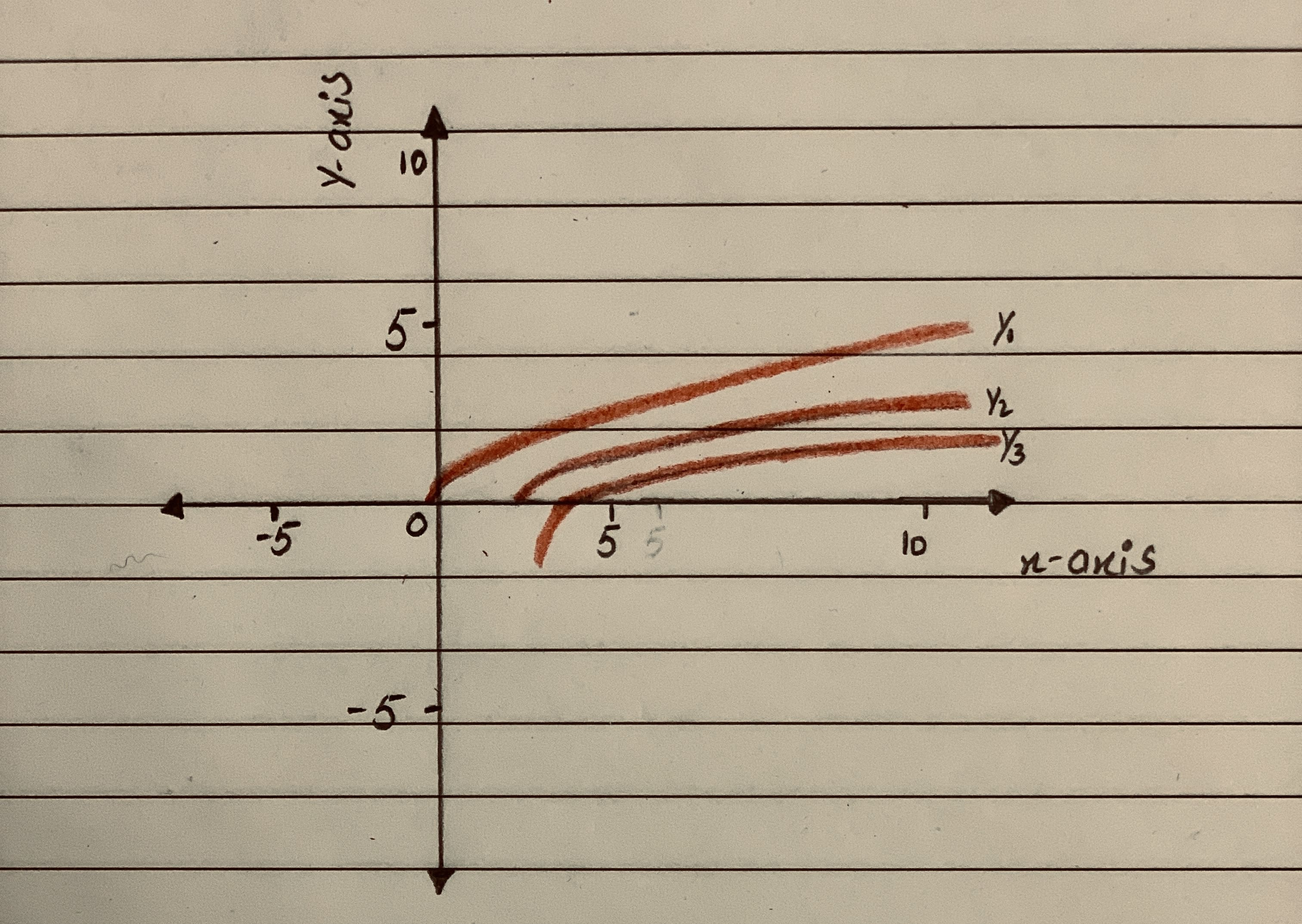# Graph the function by hand, not by plotting points, but by starting with the graph of one of the standard functions and then applying the appropriate transformations.y=sqrt(x - 2) - 1Yulia 2021-01-19 Answered

Graph the function by hand, not by plotting points, but by starting with the graph of one of the standard functions and then applying the appropriate transformations.
$y=\sqrt{x-2}-1$

You can still ask an expert for help

• Questions are typically answered in as fast as 30 minutes

Solve your problem for the price of one coffee

• Math expert for every subject
• Pay only if we can solve itBertha Stark

To determine:
Graph of $y=\sqrt{x-2}-1$ by applying appropriate transformation.
Firstly, we will draw graph of $y=\sqrt{x}$ which is given by:Y1
now, we will draw graph of $y=\sqrt{x-2}$ by shifting the graph of $y=\sqrt{x}$ two units towards right.
So, the graph of $y=\sqrt{x-2}$ is as follows:Y2
Now, we will draw graph of $y=\sqrt{x-2}-1$ by shifting the graph of $y=\sqrt{x-2}$ one unit downward along y axis.
The graph of $y=\sqrt{x-2}-1$ is given by:Y3